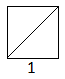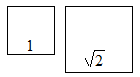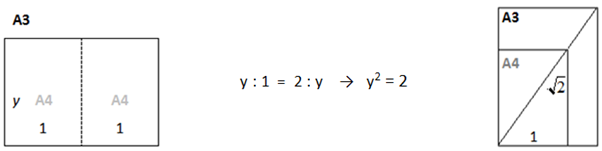# The Five-fold Path to Mathematical Wisdom via @CambridgeMaths

In an earlier post, we observed that mathematics is not simply defined by objects but by connections between objects. These connections then become new objects and then there are connections between these, and mathematics soon metamorphoses into an untameable and ever-expanding beast! Here we present a five-fold path to attempt to tame this beast. The idea is to consider a mathematical object or problem from five perspectives, and weave these together to harness the beast.

This is a re-blog post by Vinay Kathotia and published with kind permission.

The original post can be found here.

###### Submit your blog post for reblogging on UKEdChat.com by clicking here.

The Algebraic (symbolic or formal) Approach

Being able to express a problem algebraically – introducing names or symbols for quantities, constructing expressions and equations that encode given information, and performing the symbolic manipulation needed to simplify/solve the problem. This underpins much of current day mathematical infrastructure, in our use of variables for unknowns, functions, and computer programming.

The Graphic Viewpoint

Ever since René Descartes established Cartesian coordinates, algebra and geometry have been inseparably entwined. The equation x2 + y2 = r2conjures a circle, y = x a straight line, and functions can be viewed as graphs. Being able to move between the algebraic and graphical realms gives a better feel for problems and can help develop methods for solving them.

But graphical methods are not just geometric analogues of algebraic approaches – pictures and animations can bring alive pages of verbal description. But they must be interpreted with caution: while they can powerfully distil and convey information, they can also blur details and conceal errors.

The Physical (or practical) Path

Mathematical problems often arise from modelling physical situations. In fact, if one is to take the Platonic view, every problem is a ‘real world’ problem – it’s just that we may first need to discover the appropriate world! Associating a practical problem with a mathematical construct enables us to move information in either direction. Integrals let us measure area, volume, population size … but equally, interpreting abstract integrals as an area, one can estimate/calculate their value and deduce additional properties. Similarly, thinking of functions of two variables as the temperature on a particular surface, or as the height above sea level, can foster better understanding.

The Computational (or algorithmic) Method

Many problems require a numerical solution or approximation. It is imperative that one be able to travel this road, i.e., compute. There is the story of a learned sage whose complete theoretical knowledge of swimming techniques couldn’t save him from drowning – although the other extreme, being able to compute without understanding, is equally avoidable. Besides other pitfalls, it would make us prime candidates for replacement by computers, a real enough fear these days. Thus, not only should we able to implement standard algorithms but we should understand how and why they work, be able to assess their outputs, and craft better algorithms.

The Fifth (quintessential) Element is simply an affirmation that we can never exhaust the manifold ways of looking at something. It could be a historical or holistic view, a fuller understanding that evolves from connecting multiple representations, or the unique insight that you bring along. The need for this final, seemingly ambiguous approach is well articulated in the following quote of Indian teacher and president, Sarvepalli Radhakrishnan (about philosophy, but it applies equally well to mathematics):

“The danger of all human occupations is present also in philosophy, the danger of accepting standard solutions and performing mechanically, through sheer laziness and inertia, the established modes of thinking. If we teach ready-made doctrines and see in any system of thought perfection and completeness, we miss the true spirit of enquiry.”

Practising one’s preaching

To clarify the above concepts we consider an example:√2. Algebraically,√2 can be seen as a solution of the equation x2 = 2 and can be related to inverse operations (the inverse of squaring). At a computational level this could mean carrying out a ‘square-rooting’ algorithm, by hand (long-division-style) or by calculator, or using continued fractions or a host of iterative schemes, including trial and improvement.At a graphical level, √2 is the length of the diagonal of a unit square. This enables us to explicitly see it and approximate it by measurement. Alternately, we can read off its value from the graph of y = x2, a parabola.A practical significance of √2 is that it is the side-length of a square that will double the area of a unit square. Better still, it is there in every A4 sheet of paper(!) as the ‘ratio’ that defines the A (B, C, …) series of paper sizes. That is, if you want a rectangle such that halving it (parallel to the shorter side) gives a rectangle similar to the original, then the length must be √2 times the width!We’ll leave the fifth approach to the next post (there isn’t enough space here), on √2 being an irrational number, which should help bring these strands together and highlight the irrational effectiveness of mathematics!

You need to or Register to bookmark/favorite this content.